Curve Fitting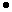GivenN points

Find Nice-looking curve through these points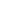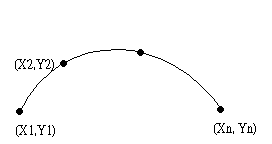Polynomial Interpolation1 polynomial of degree N-1 matches pointsComplicated,fluctuated,slowSpline Interpolationfrom mechanicspiece together N-1 cubic curves together smoothly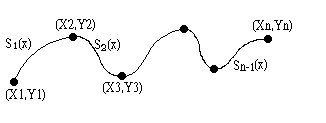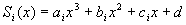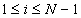(1)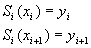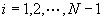(2)(3)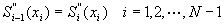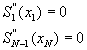4N-4 unknowns4N-4 equationsGaussian Elimination can doBetter approachLet the curves be S as described as fallows,Curse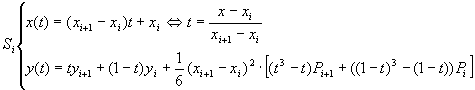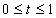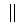we have the properties: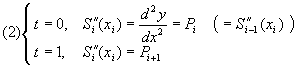to show how (2) stands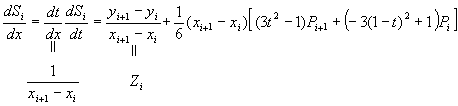so,, such that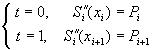and note that: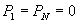Spline curveWith the two properties above, all we have to do is to determine Pi through the first differential equations: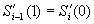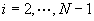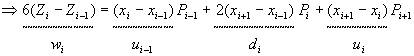which leads us to simple matrix computation.For Example, let n = 7, we can construct a matrix bellow: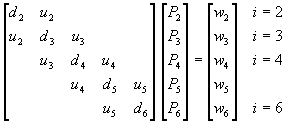Solve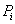, then plug them to Si to finish the approach.Time complexity: T=O(N)